4WX Internet Search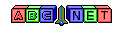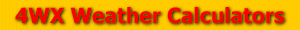# Virtual Temperature

From the user, an air temperature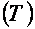, a dewpoint temperature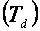, and a station pressure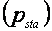are given.

To calculate the virtual temperature, the temperatures must be converted to units of degrees Celsius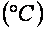and the station pressure must be converted to units of millibars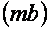To see how to convert these values, see the links below:

Then, the virtual temperature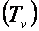can be calculated using the formula below: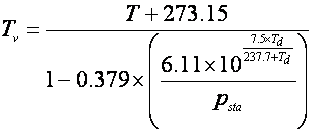The virtual temperature answer will be in units of Kelvin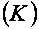, but virtual temperature can be converted to other units using the link above.

Back to 4WX Weather Calculators index page

4WX Calculators Credit and disclaimer